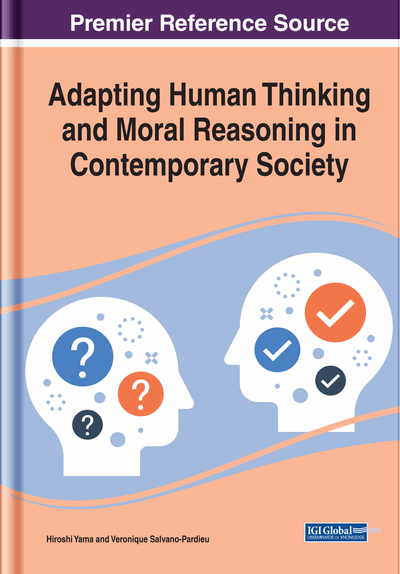# What Society Can and Cannot Learn From Coherence: Theoretical and Practical Considerations

Niki Pfeifer, Andrea Capotorti
DOI: 10.4018/978-1-7998-1811-3.ch008
OnDemand:
(Individual Chapters)
Available
\$37.50
No Current Special Offers

## Abstract

Society is facing uncertainty on a multitude of domains and levels: usually, reasoning and decisions about political, economic, or health issues must be made under uncertainty. Among various approaches to probability, this chapter presents the coherence approach to probability as a method for uncertainty management. The authors explain the role of uncertainty in the context of important societal issues like legal reasoning and vaccination hesitancy. Finally, the chapter presents selected psychological factors which impact probabilistic representation and reasoning and discusses what society can and cannot learn from the coherence approach from theoretical and practical perspectives.
Chapter Preview
Top

## Introduction

Society is facing uncertainty on a multitude of domains and levels: usually, reasoning and decisions about political, economic, or health issues have to be made under uncertainty. Probability theory allows for modeling belief states of incomplete and uncertain knowledge. Among various approaches to probability, the authors advocate the coherence approach, which goes back to Bruno de Finetti (see, e.g., 1937, 1974). The introduction presents a brief informal description of the theory of coherence. Contrary to standard approaches to probability, the coherence approach does not presuppose a definition on a complete and exhaustive mathematical structure: rather, it works with partial assessments of just those events which are of interest. This feature makes the coherence approach to probability psychologically appealing: it is plausible to assume that people draw inferences directly without storing and processing rich and complex mathematical structures like algebras. Moreover, conditional probability—which is the best predictor for human reasoning about uncertain conditionals—is primitive in the coherence approach. Therefore, probabilities can be assigned directly without building the fraction of the joint probability (i.e., the probability of the conjunction of the antecedent and the consequent) and the marginal probability (i.e., the probability of the antecedent). In everyday contexts, for example, people can assess their degree of belief in a conditional like “if Max enters the train at 6 o'clock, then Max will be home at 7 o'clock” directly, without firstly forming and secondly dividing the probability of the conjunction “Max enters the train at 6 o'clock and Max will be home at 7 o'clock” by the probability of the antecedent “Max enters the train at 6 o'clock”. Therefore, human reasoning under uncertainty is modeled more naturally by the coherence approach compared to standard approaches to probability. Furthermore, coherence allows for imprecise probabilities (i.e., interval-valued probabilities): this feature becomes relevant when there is not enough information to give point-valued assessments. Also, conclusions may be imprecise even if the premises are precise: for example, from the precise premises P(Max enters the train at 6 o'clock)=.8 and P(Max will be home at 7 o'clock given Max enters the train at 6 o'clock)=.9 only the imprecise conclusion that .72 ≤ P(Max will be home at 7 o'clock)≤ .92 is coherent can be drawn (see, e.g., Pfeifer & Kleiter, 2006, 2009). Moreover, the coherence approach shows its full potential when the premise set does not only contain probabilistic information but also logical information about logical interconnections (like logical implications, incompatibilities, exhaustiveness, etc.).

## Key Terms in this Chapter

Availability Heuristic: The evaluation of the probability of an event is derived from the ease with which instances of the event are retrieved from memory.

Belief Bias: Whether an argument is evaluated as valid depends only on whether the conclusion is believable (irrespective of the premise set).

Fake News: Wrong information communicated as true information.

Vaccine Hesitancy: A reluctance or refusal to be vaccinated or to have one’s children vaccinated.

Probability Assessment: A numerical evaluation of uncertainty on a finite set of events, not necessarily endowed with any mathematical structure but interconnected by logical relations, like implications, incompatibilities, equivalences, etc.

Algebra: Mathematical structure of a set of events, which contains the tautology and which is closed under finite disjunctions and negation. An algebra is also closed under finite conjunctions by de Morgan’s laws. If an algebra is closed under infinite disjunctions and conjunctions, it is called “??-algebra” (read: sigma-algebra).

Dutch Book: A hypothetical combination of symmetric bets, where the player or the banker can switch each other’s bets, such that it will lead to a sure win (or a sure loss).

Coherence: A subjective approach to probability which means semantically to avoid Dutch books. Syntactically, coherence means the solvability of a specific linear system that reflects the existence of at least one standard probability distribution which is compatible with the initial probability assessment.

Argument: Linguistic structure consisting of the premise set (i.e., known or assumed information) and the conclusion (what is supposed to follow from the premises).

## Complete Chapter List

Search this Book:
Reset